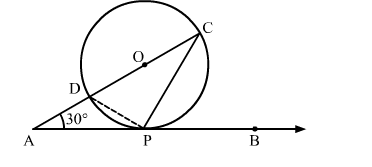# In the given figure, O is the centre of the circle. AB is the tangent to the circle at the point P.`
Question:

In the given figure, O is the centre of the circle. AB is the tangent to the circle at the point P. If  ∠PAO = 30  then ∠CPB + ∠ACP is equal to
(a) 60
(b) 90
(c) 120
(d) 150Solution:

We know that a chord passing through the centre is the diameter of the circle.
∵∠DPC = 90    (Angle in a semi circle is 90)
Now, In △CDP
∠CDP + ∠DCP + ∠DPC = 180            [Angle sum property of a triangle]
⇒ ∠CDP + ∠DCP +  90 = 180
⇒ ∠CDP + ∠DCP = 90
By using alternate segment theorem
We have ∠CDP = ∠CPB
∴∠CPB + ∠ACP = 90
Hence, the correct answer is option (b).